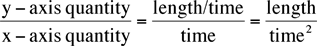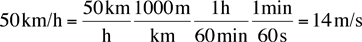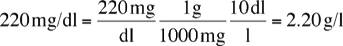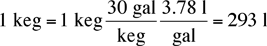Meiji Techno America Explanation: Dimensions, Units and Conversions

Science depends on measured numbers, most of which have units. The following sections should help you understand what units are, how they are used, and how they are converted from one system to another.

Dimensions vs units

It is fairly easy to confuse the physical dimensions of a quantity with the units used to measure the dimension. We usually consider quantities like masslengthtime, and perhaps charge and temperature, as fundamental dimensions. We then express the dimensions of other quantitieslike speed, which is length/time, in terms of the basic set. The point is that every quantity which is not explicitly dimensionless, like a pure number, has characteristic dimensions which are not affected by the way we measure it. As we will see later, this provides a useful check on any calculations we do.

Units give the magnitude of some dimension relative to an arbitrary standard. For example, when we say that a person is six feet tall, we mean that person is six times as long as an object whose length is defined to be one foot. The standard size chosen is, of course, entirely arbitrary, but becomes very useful for comparing measurements made in different places and times. Several national laboratories are devoted to maintaining sets of standards, and using them to calibrate instruments.

In contrast to dimensions, of which only a few are needed, there is a multitude of units for measuring most quantities. You have probably heard of lengths measured in inches, feet, miles, meters, centimeters and kilometers, but there are also furlongs, rods, Angstroms, nautical miles, parsecs and many others. It is, therefore, always necessary to attach a unit to the number, as when giving a person’s height as 5 feet 9 inches, or as 175 cm. Without units, a number is at best meaningless and at worst misleading to the reader.

Dimensionless quantities should be easier, in that they do not have units at all, but in some ways they are more complicated. Some examples:

• Ratios: The Mach number is defined as the ratio of vehicle speed to the speed of sound at a specific altitude. Since it is a ratio, it is a pure number, with neither dimensions nor units. Similarly, the chemist’s mole is the ratio of the number of molecules present to Avogadro’s number. To be meaningful, the ratio has to carry its title with it, as in Mach 0.8 for a passenger jet, or 0.15 mole for a pile of some chemical.

• Angles: To a mathematician, the magnitude of an angle is the ratio of subtended arc length to radius on a circle. This definition gives numbers from 0 to 2 p . Confusion arises because people have also been dividing circles into 360 parts from dim antiquity. Scientists usually use the mathematical definition, but not always, so any angular measurement needs to be labeled. Use radians for the mathematical definition, and degrees for the customary. Be sure your calculator is set for the right one, usually radians, when evaluating trig functions.

• Numbers: A simple number, like the total number of trees on campus, is clearly not difficult, you just need to specify what you are counting.

Finally, and unfortunately, there are some customary units that have the wrong dimensions for the quantity they purport to measure. Two cautionary examples should suffice:

• Mass-energy: Physicists often refer to the mass of the electron as 0.51 MeV, but the MeV is a unit of energy, not mass. The connection is through the famous E = mc2, assuming that the entire mass were to be converted to energy.

• Pressure: Pressure has dimensions of force/area, but blood pressure is measured in mmHg, which seems to be a length. The unit actually refers to the pressure required to support a column of mercury 1 mm high. Conversion to a true pressure requires knowing the density of mercury and the gravitational force.

The moral is for both the reader and the author to be very aware of what units are being used

Conventional choices

The choice of units depends purely on convenience and custom, so it is always necessary to specify the units with any number. The next user of whatever report you are preparing may be able to deduce the dimensions of quantities, but it is hopeless to expect a reader to guess correctly what units you used if you do not report them.

Preferred units

Most fields have preferred units for their own uses. Using preferred units will facilitate communication and minimize errors. Again, some examples may help:

Mariners and aviators measure distances in nautical miles. The general public in the US, and NASA, use statute miles. (The difference once caused a laser-ranging experiment on the shuttle to fail.)

Mariners and aviators measure speed in knots, namely nautical miles per hour, whereas the rest of the US uses miles/hour for vehicles. Most of the rest of the world uses kilometers/hour.

Scientists usually specify speeds in meters/second, but a biologist studying snail motion might prefer millimeters/second.

Chemists often express concentrations in moles/liter or grams/liter, but medical laboratories frequently use milligrams/deciliter.

Thermal quantities may be measured in calories by a chemist or biologist, in “large calories” by a nutritionist, in Joules by a physicist, or BTU by a heating engineer.

Despite the diversity, some generalizations are possible. Electrical quantities are almost always measured in standard metric units, such as volts, amps and ohms. For mechanical quantities, physical scientists tend to use meters, kilograms and seconds (“MKS units”), or units derived from those. Biologists and chemists seem to prefer centimeters, grams and seconds (“cgs units”). Engineers in the US may need to employ both metric units and British customary units, depending on their subject. The moral is, always find out what units are appropriate for the task at hand, and express your results accordingly.

Prefixes and suffixes

It is often convenient to use units which are a multiple or fraction of the basic unit. The metric system, formally known as the International System of Units, defines a number of prefixes to denote powers of ten. The current official set is tabulated here:

Prefixes used by the International System of Units (SI)

 Prefix Factor Symbol Prefix Factor Symbol deci 10-1 d deca 10 da centi 10-2 c hecto 102 h milli 10-3 m kilo 103 k micro 10-6 µ mega 106 M nano 10-9 n giga 109 G pico 10-12 p tera 1012 T femto 10-15 f peta 1015 P atto 10-18 a exa 1018 E

The larger or smaller unit is denoted by attaching the prefix to the unit name. For example, a centimeter is 10-2 meter, a millivolt is 10-3 volt, and a megawatt is 106 watts.

Dimensional Consistency

Dimensions and units must be handled consistently in any algebraic calculation. To be added, two quantities must have the same dimensions and units. (Adding a volume and a mass is guaranteed to be wrong.) The factors in a multiplication or division may have different units, and the combined quantity will have units of the product or ratio of the factors. Equations involving physical quantities must have the same dimensions on both sides, and the dimensions must be the correct ones for the quantity calculated. The units on both sides will usually also be the same, and must be at least equivalent and correct.

Verifying dimensional consistency is often called “checking the units,” and is a powerful technique for uncovering errors in calculations. For purposes of checking consistency, dimensions or units may be considered algebraic quantities. Some examples of this procedure are:

• Density is defined as the ratio of mass to volume, and must have dimensions of mass/(length)3, with corresponding units.

• Checking dimensions for the famous formula E = mc2

(energy) = (mass) (speed)2
(force) (length) = (mass) (length/time)2
(mass) (acceleration) (length) = (mass) (length)2/(time)2
(mass) (length/time2) (length) = (mass) (length)2/(time)2
(mass) (length)2/(time)2 = (mass) (length)2/(time)2

which agrees as expected. We could have gotten the same result using units of joules, kg and m/s.

• A student making artificial sea water dissolves 13.1 gm of NaCl in 450 ml of distilled water, and calculates the resulting concentration as 0.0291 gm/ml. A standard handbook claims that seawater has 29.54 gm/l of NaCl. Comparing units, the student recalculates the concentration as 13.1 gm/ 0.45 l = 29.1 gm/l, and notes that the units are now the same and the magnitude is sufficiently close.

• A radar gun is used to obtain the speed of a car as it accelerates from a stop sign. A graph of speed (y-axis) vs time (x-axis) is a straight line, so the student computes a slope expecting to find the constant acceleration. The slope of this line is a ratio ofwhich is an acceleration, as expected. If the axes had been reversed, plotting time vs speed, the student would have gotten the wrong dimensions and recognized an error.

Unit conversions

It is often necessary to change units in order to combine measurements made with different instruments or express a result in customary form. The procedure is very simple if the units are again handled as algebraic quantities. Just multiply the starting units by properly chosen factors of one until the desired units are left. A couple of examples will show how it is done.

• Express a speed of 50 kilometers per hour as meters per secondNote how the units ‘cancel’ in the middle terms, leaving only the desired quantities

• Convert a concentration of 220 mg/dl to grams/literThis one is easy, since metric units involve only factors of ten, but being systematic means you get all the tens in the right places

• A European acquaintance asks how much beer is in a “full keg”. This is just a volume conversion, since Europeans measure fluids in liters, but you will probably need a reference to get the factors:Sometimes what appears to be a units conversion isn’t just a change of units, but an actual change in the quantity expressed. For example, to go from a solution concentration in g/l to moles/l requires a knowledge of the molecular weight of the dissolved substance since you are changing from mass to number of molecules. To go from a pressure specified as mmHg to the proper metric unit of Nt/m2 you need to understand how the unit is defined, the density of mercury and the strength of the gravitational force on Earth. (Better yet, find a good table of conversion factors for this one).